# Verification Hindcast

### ACC

Addresses the question: How well did the forecast anomalies correspond to the observed anomalies?

Range: -1 to 1. Perfect score: 1.

Characteristics: Measures correspondence or phase difference between forecast and observations, subtracting out the climatological mean at each point, C, rather than the sample mean values. The anomaly correlation is frequently used to verify output from numerical weather prediction (NWP) models. AC is not sensitive to forecast bias, so a good anomaly correlation does not guarantee accurate forecasts. Both forms of the equation are in common use -- see Jolliffe and Stephenson (2012) or Wilks (2005) for further discussion.

In the example above, if the climatological temperature is 14 C, then AC = 0.904. AC is more often used in spatial verification.

### RMSE

Answers the question: What is the average magnitude of the forecast errors?

Range: 0 to ∞. Perfect score: 0.

Characteristics: Simple, familiar. Measures "average" error, weighted according to the square of the error. Does not indicate the direction of the deviations. The RMSE puts greater influence on large errors than smaller errors, which may be a good things if large errors are especially undesirable, but may also encourage conservative forecasting.

In the example above, RMSE = 3.2 C

The root mean square factor is similar to RMSE, but gives a multiplicative error instead of an additive error.

### MSSS

The binary event can be defined as the occurrence of one of two possible categories when the outcome of the LRF system is in two categories. When the outcome of the LRF system is in three (or more) categories, the binary event is defined in terms of occurrences of one category against the remaining ones. In those circumstances, ROC has to be calculated for each possible category.

3.3.1 MSSS for non-categorical deterministic forecasts

Let xij and fij(i=1, ..., n) denote time series of observations and continuous deterministic forecasts respectively for a grid point or station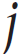over the period of verification(POV). Then, their averages for the POV,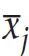and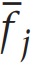and their sample variances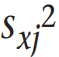and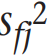are given by:

The mean squared error of the forecasts is:

For the case of cross-validated (see section 3.4) POV climatology forecasts where forecast/observation pairs are reasonably temporally independent of each other (so that only one year at a time is withheld), the mean squared error of ‘climatology’ forecasts (Murphy, 1988) is:

The Mean Squared Skill Score (MSSS) for j is defined as one minus the ratio of the squared error of the forecasts to the squared error for forecasts of ‘climatology’:

For the three domains described in Sec. 3.1.1 it is recommended that an overall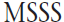be provided. This is computed as:

where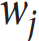is unity for verifications at stations and is equal to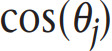, where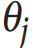is the latitude at grid pointon latitude-longitude grids. For either MSSSj or, a corresponding Root Mean Squared Skill Score (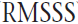) can be obtained easily from:

MSSSj for forecasts fully cross-validated (with one year at a time withheld) can be expanded (Murphy, 1988) as:

where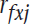is the product moment correlation of the forecast sand observations at point or station.

The first three terms of the decomposition of MSSSj are related to p hase errors (throughthecorrelation), amplitude errors (through the ratio of the forecast to observed variances)and overall bias error, respectively, of the forecasts. These terms provide the opportunity for those wishing to use the forecasts for input into regional and local forecasts to adjust or weight the forecasts as they deem appropriate. The last term takes into account the fact that the ‘climatology’ forecasts are cross-validated as well.

Note that for forecasts with the same amplitude as that of observations (second term unity) and no overall bias (third term zero), MSSSj will not exceed zero(i.e.the forecasts squared error will not be less than f or‘climatology’) unless rfxj exceeds approximately0.5.

The core SVSLRF requires grid-point values of the correlation, the ratio of the square roots of the variances, and the overall bias i.e.

.

In addition it is recommended that grid-point (j) values of the following quantities are provided:

As an additional standard against which to measure forecast performance, cross-validated damped persistence (defined below) should be considered for certain forecast sets. A forecast of ordinary persistence, for a given parameter and target period, stands for the persisted anomaly (departure from cross-validated climatology) from a period immediately preceding the start of the lead time for the forecast period (see Figure 1). This period must have the same length as the forecast period. For example, the ordinary persistence forecast for a 90-day period made 15 days in advance would be the anomaly of the 90-day period beginning 105 days before the target forecast period and ending 16 days before. Ordinary persistence forecasts are never recommended as a standard against which to measure other forecasts if the performance or skill measures are based on squared error, like herein. This is because persistence is easy to beat in this framework.